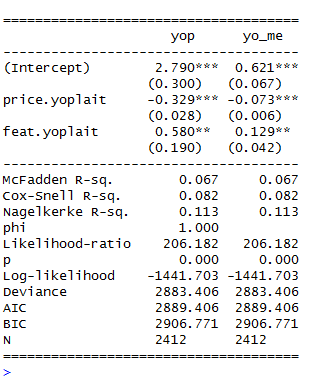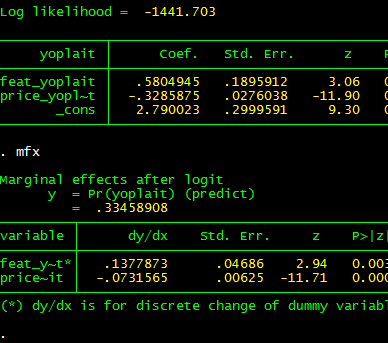## Thursday, May 19, 2011

### Porting Stata-like Marginal Effects to LaTeX

The TimeSeriesIreland Blog posted an excellent start on a function that automatically computes marginal effects for probit and logit models. As I have always wanted an easy-to-use function that computes and reports marginal effects in R, I was elated to see the function and I couldn't wait to use it.

My initial impression when I saw the post was: "I should write an mtable() extension for that to go with my other extensions." With this in mind, I started familiarizing myself with the code and I contacted the author to make sure he was fine with me extending his code (and adding it to my package with mtable() extensions). As he was fine with my goal of extending the function, here is a post where I introduce you to a new extension to mtable().

Important Caveat: At this point, the function does not do the technically "right" thing (delta method) to obtain the standard errors on the marginal effects. Instead, it supposes that the z-score will remain constant across the transformation, which isn't quite right. Even though it isn't perfect, in most of the examples I have tried, the results are almost identical to Stata output. You tend to get more divergence from the Stata solution for dummy variable regressors (where Stata computes a discrete jump rather than a derivative).

One would think that an extension of mfx() would use mfx(), but that didn't turn out to be the easy way to go. After many unsuccessful attempts (more about this in a future post; I learned some things worth sharing), here's the solution I landed on.

First, define an "mfx" object with a function that changes the class attribute of a fitted glm object to. Here's code to define a function to do this.

Second, make sure you have the memisc library loaded with the command library(memisc) -- or library(tonymisc), which loads memisc among other excellent packages.

Third, until I get the kinks worked out and port this into my package, you'll have to run code to define a getSummary.mfx() method to extend mtable. Here's some code that will do this.

Now, I suppose you want to know how this extension works. Here's an example that uses some data from the wonderful Ecdat library (a great source for Econometrics data sets).

Here's the output to R's console. Note that feat.yoplait is a dummy variable for whether Yoplait had a featured advertisement that particular week. Based on my caveat, you wouldn't expect the output to be identical to Stata.And, as you can see (compare with Stata output below), it isn't exactly the same, but if you are trying to understand the qualitative importance of your estimates, it isn't too bad.Two bugs (quirks, kludges) remain: (1) I want to get the standard errors precisely right, (2) I want to convince mtable() to stop reporting the intercept for the marginal effects (as the intercept doesn't change, it can't have a marginal effect!). Even before I get around to this, I hope this code will have a positive marginal effect.

---

Update: The original post to which this post refers is no longer available because the TimeSeriesIreland Blog appears to have been taken down. I leave this post up subject to two caveats: (1) the standard errors are not quite right for the marginal effects and (2) the marginal effects for dummy regressors are not computed for a discrete jump from 0 to 1. Otherwise, you may find this tool useful.

Subject to the caveats in this post, if you want access to this function and a related function that is more summary()-like, I have included it in my CRAN-contributed package tonymisc (see the tab at the top of this page).

#### 1 comment:

1.Hi, I'm a French PhD student an I work on a tobit model with the 2steps Heckman method (function "heckit2fit" from the "sampleSelection" package). I need to calculate the marginal effects for the selection equation (probit) and for the outcome equation. For the selection equation I found a mfx function on a working paper that seems to work well (http://www.ucd.ie/t4cms/WP11_22.pdf) but for the outcome equation I find nothing that could give the marginal effects of variables that are used in both equations as Stata does. Can you help me with that?
Another point : Whether I use the mfx fucntion decribed in the working paper I mentionned above or my own script for the calculation of the probit marginal effects, I find similar marginal effects... but they both are 10 times inferior to the marginal effects given by stata (I had the opportunity to compare thanks to a friend who has Stata)...I don't understand where these differences come from, the only thing I notice is that "rho" of my heckit model is absolutely not the same with Stata and with R...
Thanks you for your help.
Laurence Delattre.
(French National Institute for Agricultural Research)